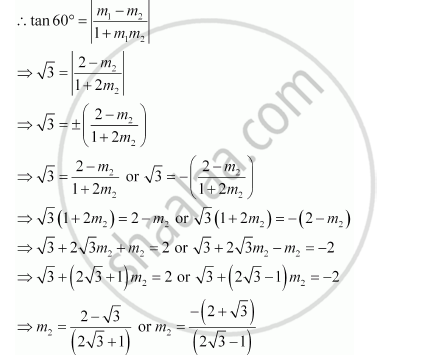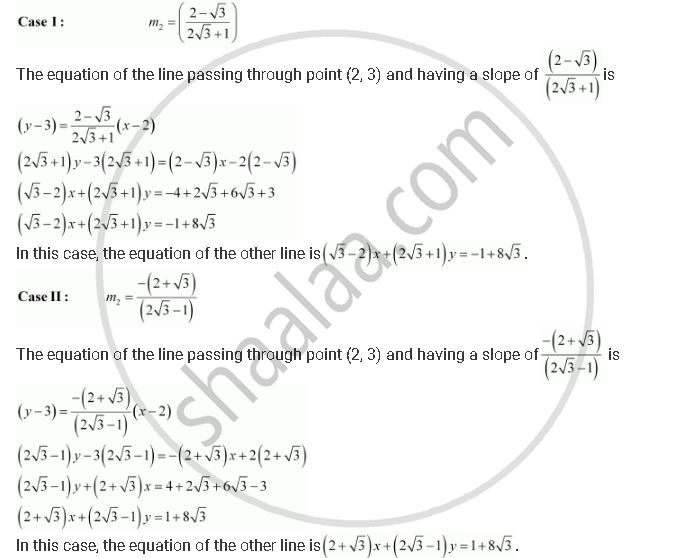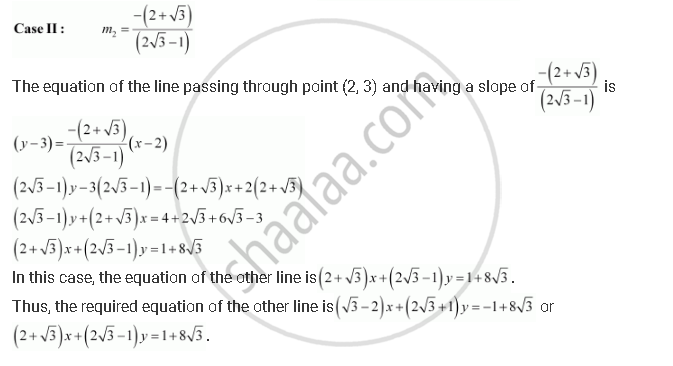CBSE (Arts) Class 11CBSE
Share

# Two Lines Passing Through the Point (2, 3) Intersects Each Other at an Angle of 60°. If Slope of One Line is 2, Find Equation of the Other Line. - CBSE (Arts) Class 11 - Mathematics

ConceptGeneral Equation of a Line

#### Question

Two lines passing through the point (2, 3) intersects each other at an angle of 60°. If slope of one line is 2, find equation of the other line.

#### Solution

It is given that the slope of the first line, m1 = 2.

Let the slope of the other line be m2.

The angle between the two lines is 60°.Is there an error in this question or solution?

#### Video TutorialsVIEW ALL 

Solution Two Lines Passing Through the Point (2, 3) Intersects Each Other at an Angle of 60°. If Slope of One Line is 2, Find Equation of the Other Line. Concept: General Equation of a Line.
S